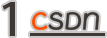``````int fun() {  int a = 2;  return &a;}void main() {  int* p = fun();  *p = 20;}
``````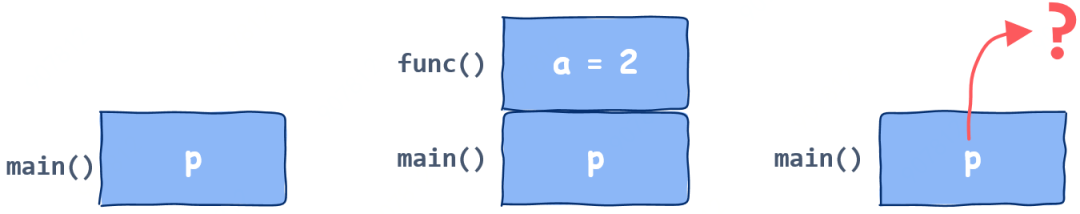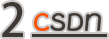``````int sum(int* arr, int len) {  int sum = 0;  for (int i = 0; i < len; i++) {      sum += *arr;      arr += sizeof(int);  }  return sum;}
``````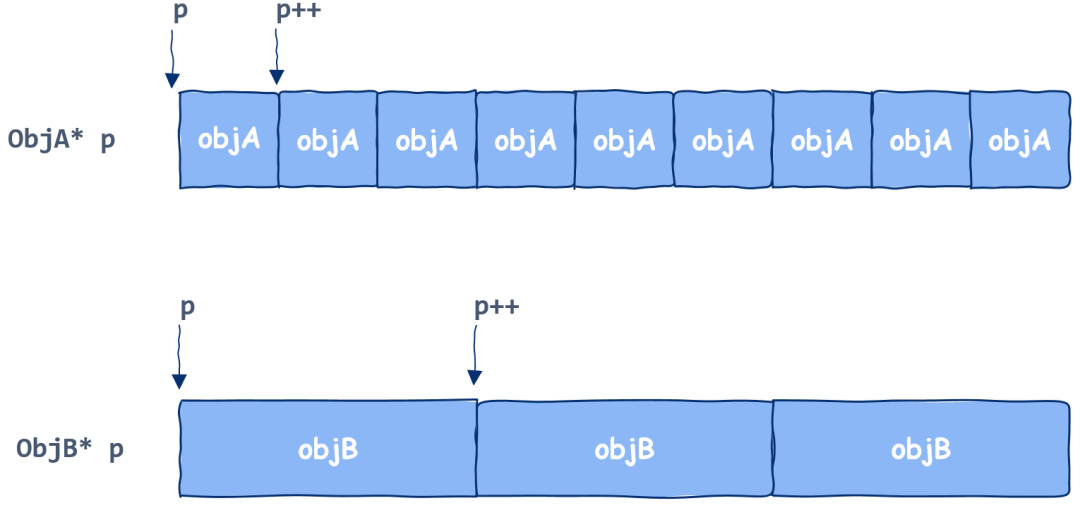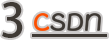C语言初学者常会犯一个经典错误，那就是从标准输入中获取键盘数据，代码是这样写的：

``````int a;scanf("%d", a);
``````

1. 如果a的值作为指针指向代码区或者其它不可写区域，操作系统将立刻kill掉该进程，这是最好的情况，这时发现问题还不算很难

2. 如果a的值作为指针指向栈区，那么此时恭喜你，其它函数的栈帧已经被破坏掉了，那么程序接下来的行为将脱离掌控，这样的 bug 极难定位

3. 如果a的值作为指针指向堆区，那么此时也恭喜你，代码中动态分配的内存已经被你破坏掉了，那么程序接下来的行为同样脱离掌控，这样的bug也极难定位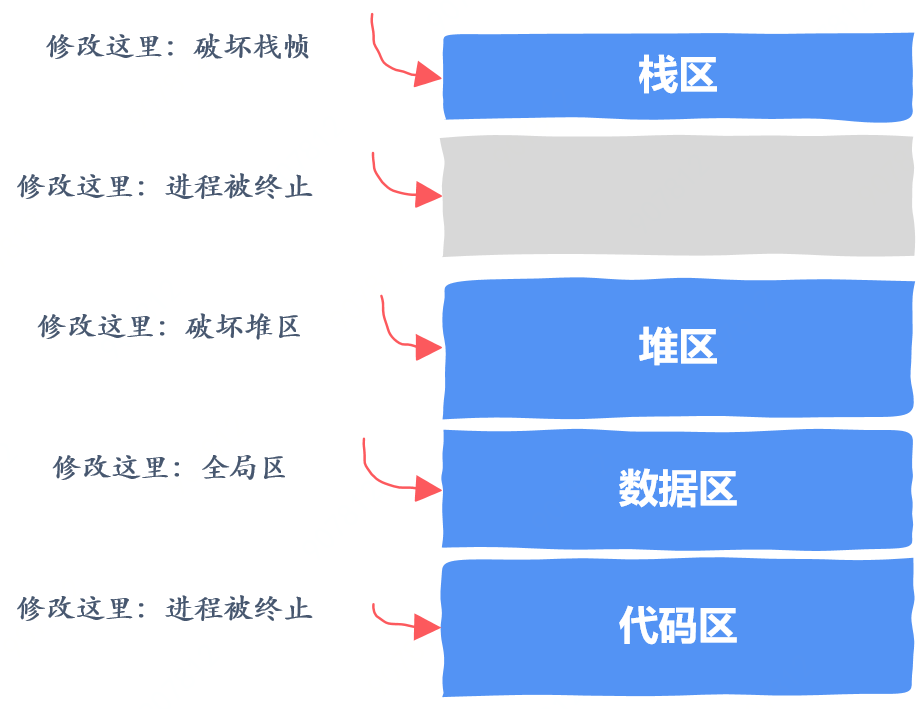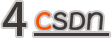``````void add() {  int* a = (int*)malloc(sizeof(int));  *a += 10;}
``````

1. 如果 malloc 自己维护的内存够用，那么 malloc 从空闲内存中找到一块大小合适的返回，注意，这一块内存可能是之前用过后释放的。在这种情况下，这块内存包含了上次使用时留下的信息，因此不一定为0

2. 如果 malloc 自己维护的内存不够用，那么通过 brk 等系统调用向操作系统申请内存，在这种情况下操作系统返回的内存确实会被初始化为0。

原因很简单，操作系统返回的这块内存可能之前被其它进程使用过，这里面也许会包含了一些敏感信息，像密码之类，因此出于安全考虑防止你读取到其它进程的信息，操作系统在把内存交给你之前会将其初始化为0。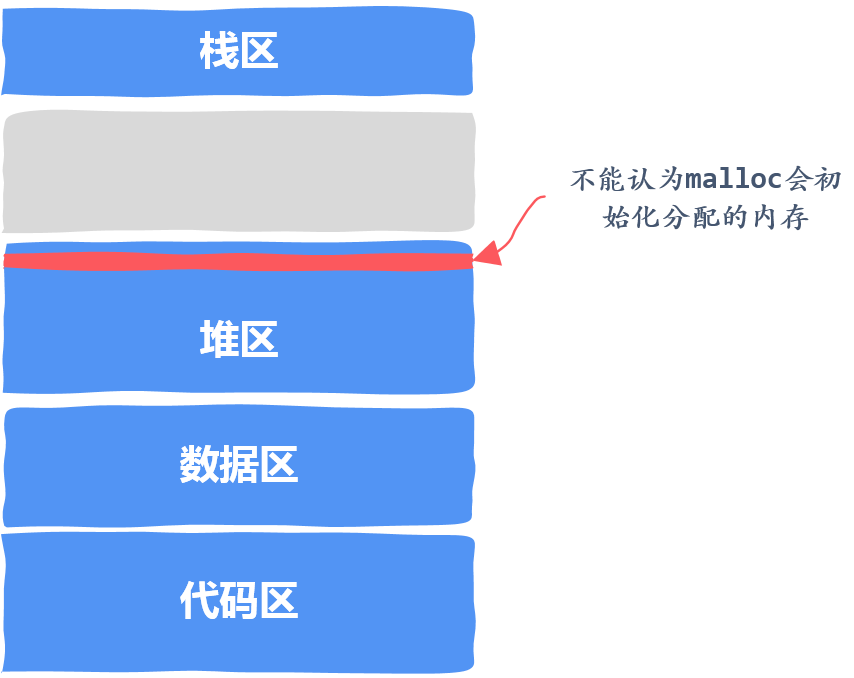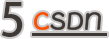``````void memory_leak() {  int *p = (int *)malloc(sizeof(int));  return;}
``````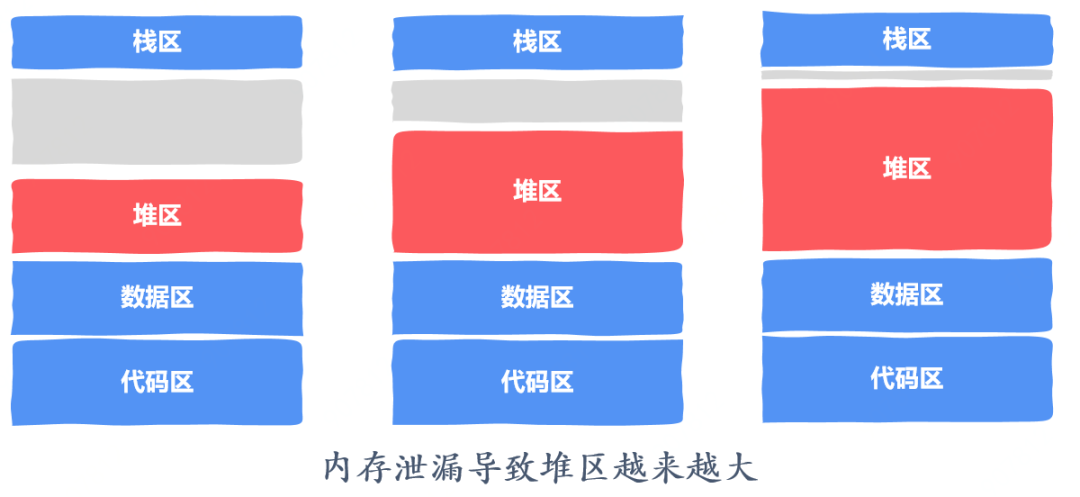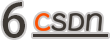``````void add() {  int* a = (int*)malloc(sizeof(int));  ...  free(a);  int* b = (int*)malloc(sizeof(int));  *b = *a;}
``````

1. 指针a指向的那块内存释放后没有被 malloc 再次分配出去，那么此时a指向的值和之前一样

2. 指针a指向的那块内存已经被 malloc分配出去了，此时a指向的内存可能已经被覆盖，那么*b得到的就是一个被覆盖掉的数据，这类问题可能要等程序运行很久才会发现，而且往往难以定位。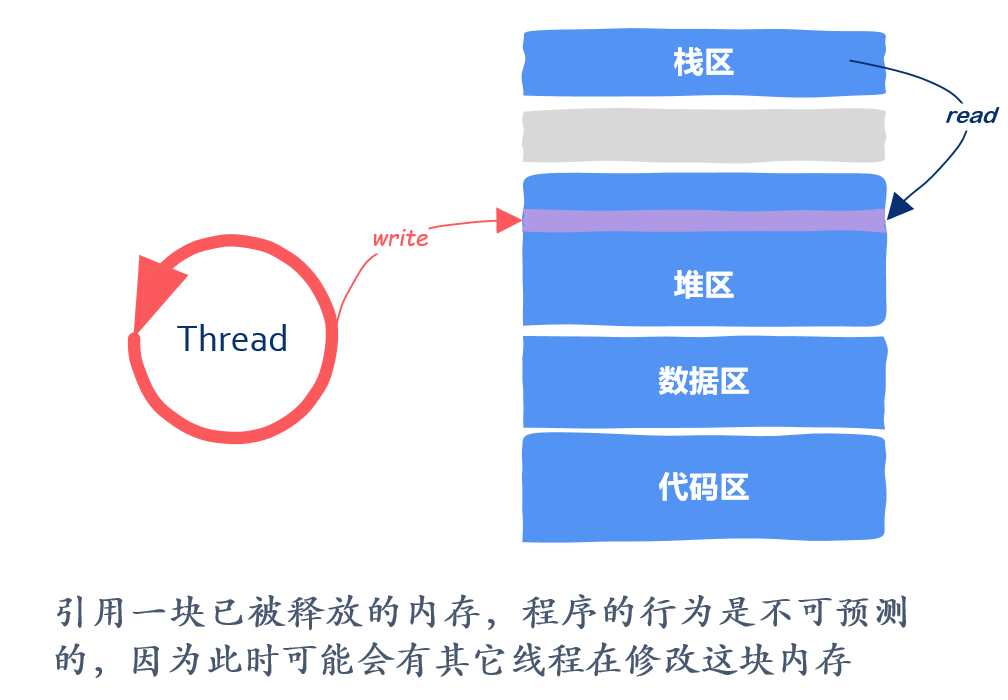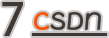``````void init(int n) {  int* arr = (int*)malloc(n * sizeof(int));  for (int i = 0; i <= n; i++) {      arr[i] = i;  }}
``````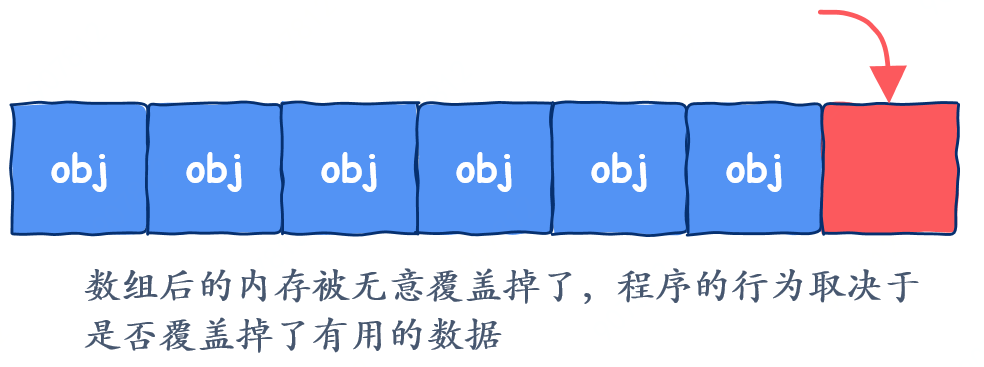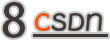###### 指针大小与指针所指向对象的大小不同
``````int **create(int n) {int i;int **M = (int **)malloc(n * sizeof(int));
for (i = 0; i < n; i++)  M[i] = (int *)malloc(m * sizeof(int));   return M;}
``````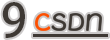``````void buffer_overflow() {char buf;
gets(buf);return;}
``````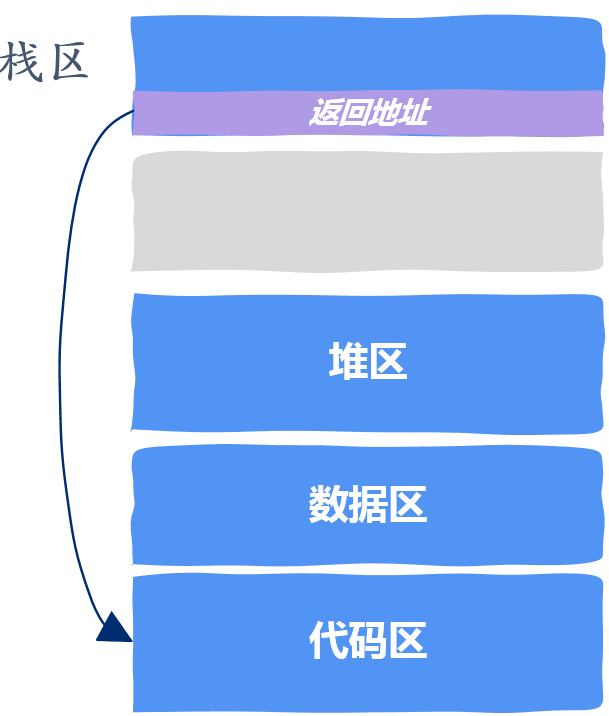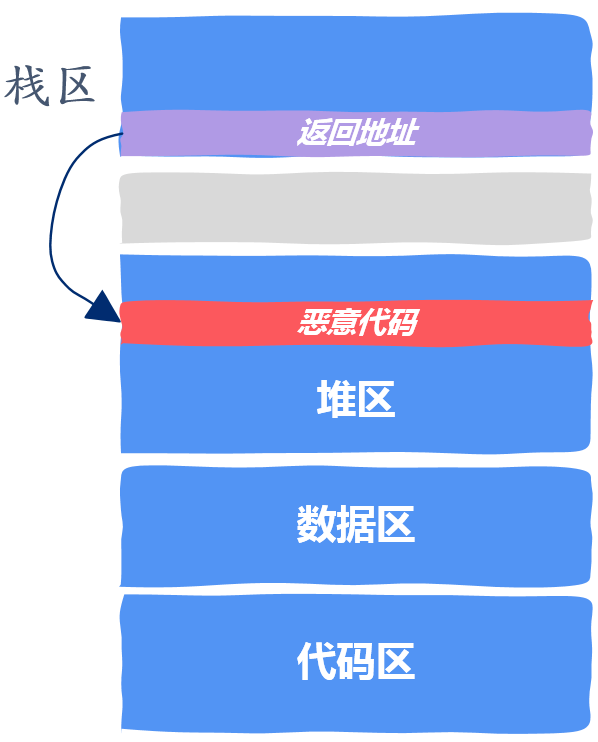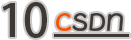``````void delete_one(int** arr, int* size) {  free(arr[*size - 1]);  *size--;}
``````

arr 是一个指针数组，这段代码的本意是要删除掉数组中最后一个元素，同时将数组的大小减一。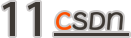``````
☞破解技术人才招聘难、培养难！CSDN 软件工程师能力认证标准正式开源开放☞源代码从 300 行到 172,000 行，它用了 23 年
☞将 Linux 移植到 M1 Mac 真的太难了！
``````20年前，《新程序员》创刊时，我们的心愿是全面关注程序员成长，中国将拥有新一代世界级的程序员。20年后的今天，我们有了新的使命：助力中国IT技术人成长，成就一亿技术人！